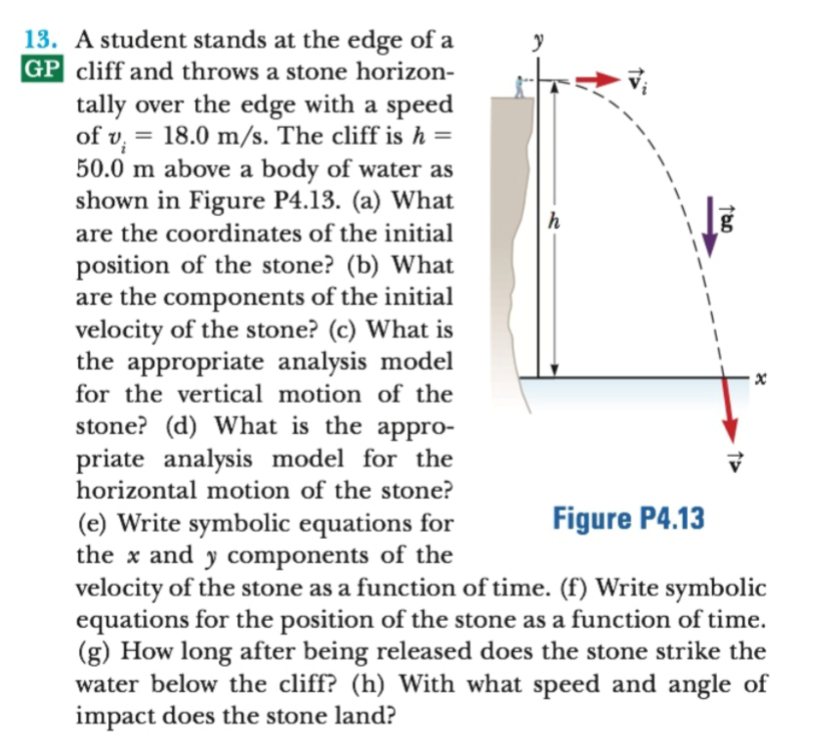# 13. A student stands at the edge of aGP cliff and throws a stone horizon-tally over the edge with a speedof v 18.0 m/s. The cliff is h =50.0 m above abody of water asshown in Figure P4.13. (a) Whatare the coordinates of the initialhposition of the stone? (b) Whatare the components of the initialvelocity of the stone? (c) What isthe appropriate analysis modelfor the vertical motion of thexstone? (d) What is the appro-priate analysis model for thehorizontal motion of the stone?Figure P4.13(e) Write symbolic equations forthe and y components of thevelocity of the stone as a function of time. (f) Write symbolicequations for the position of the stone as a function of time(g) How long after being released does the stone strike thewater below the cliff? (h) With what speed and angle ofimpact does the stone land?

Question

question in the imagehelp_outlineImage Transcriptionclose13. A student stands at the edge of a GP cliff and throws a stone horizon- tally over the edge with a speed of v 18.0 m/s. The cliff is h = 50.0 m above a body of water as shown in Figure P4.13. (a) What are the coordinates of the initial h position of the stone? (b) What are the components of the initial velocity of the stone? (c) What is the appropriate analysis model for the vertical motion of the x stone? (d) What is the appro- priate analysis model for the horizontal motion of the stone? Figure P4.13 (e) Write symbolic equations for the and y components of the velocity of the stone as a function of time. (f) Write symbolic equations for the position of the stone as a function of time (g) How long after being released does the stone strike the water below the cliff? (h) With what speed and angle of impact does the stone land? fullscreen
check_circle

Step 1

­­­­

As per our instructions we are supposed to answer only three sub parts of a question , please post the remaining sub-parts as separate question.

Part A:

Co-ordinates of initial position of the stone:

X – coordinate of the initial position = 0 meters

Y – coordinate of the initial position = 50 meters

(x,y) = ( 0, h) = (0 ,50)

Step 2

Part  B:

Components of initial velocity of the stone

X- component of velocity Vx = 18 m/s .

Y – component of velocity Vy = 0 m/s.

Step 3

Part C:

Since there is no initial vertical velocity for the stone , it is a kind of free fall motion .

Approximate analysis m...

### Want to see the full answer?

See Solution

#### Want to see this answer and more?

Solutions are written by subject experts who are available 24/7. Questions are typically answered within 1 hour.*

See Solution
*Response times may vary by subject and question.
Tagged in

### Physics